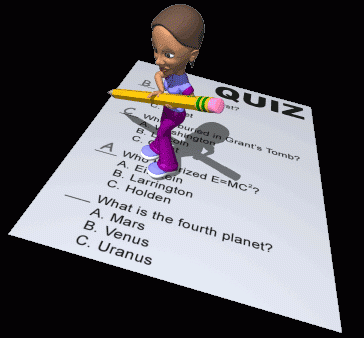Ultrasound Physics: Interaction With Soft Human Tissue

20 Questions | Total Attempts: 380SettingsUltrasound Physics and Instrumentation Examinationby: Emmanuel Pelingon, RVTThis quiz is created for practice purposes of those who are planning to take their Ultrasound Physics Exam. INSTRUCTIONS: read and understand the questions carefully. Tick the icon that corresponds to your answer of choice. You have up to 20 minutes to answer the questions. This quiz has 20 questions with total score of 100 points. Each number weighs certain points depending on the type and difficulty of the question. Passing grade is 70%. Bawal tanga at engot! Nakakamatay! Good luck!

• 1.
Ultrasound interacts with human soft tissue in several predictable ways that allow engineers to design instruments that provide diagnostic information that forms the basis of medical sonography. Which of the following parameters is not considered by engineers?
• A.

Attenuation

• B.

Reflection

• C.

Amplification

• D.

Interference

• 2.
Attenuation rate in the human body (roundtrip)
• A.

1 dB/cm/MHz

• B.

0.5 dB/cm/MHz

• C.

1. dB/cm/Hz

• D.

0.5 dB/cm/Hz

• 3.
It is the bending of sound waves at the interface or boundary of media with different acoustic impedance.
• A.

Refraction

• B.

Reflection

• C.

Reverberation

• D.

Diffraction

• 4.
Velocity times density equals?
• A.

Impedance

• B.

Intensity

• C.

Duty factor

• D.

Mass

• 5.
What is necessary to calculate distance to a reflector?
• A.

Propagation speed and round-trip time

• B.

Density and round-trip time

• C.

Density and pulse length

• D.

Attenuation rate and pulse length

• 6.
The degree of attenuation is dependent on?
• A.

Frequency and propagation speed

• B.

Distance and angle of incidence

• C.

Wavelength and amplitude

• D.

Frequency and distance

• 7.
What is scattering?
• A.

Bending of sound beam in any direction

• B.

Redirection of sound beam in many directions

• C.

Conversion of sound energy to heat energy

• D.

None of the above

• 8.
A reflector that is small compared to the wavelength of the transmitted frequency will produce,
• A.

Multiple artifact

• B.

Snell's scattering

• C.

Huygen's principle

• D.

Rayleigh scattering

• 9.
Which of the following physical occurrences is described in Snell's law?
• A.

Reflection

• B.

Diffraction

• C.

Refraction

• D.

Displacement

• 10.
Reflection at tissue interface depends primarily on
• A.

Impedance

• B.

Bandwidth

• C.

Beam profile

• D.

Velocity difference

• 11.
The intensity reflection coefficient depends on acoustic impedance mismatch.
• A.

True

• B.

False

• 12.
which of the following statements describes reflection? I. the amount of reflected energy depends on the difference in the acoustic impedance of two media to which ultrasound passes through. II. it is the bending of wave when it passes through a medium with different density . III.  bouncing back of the wave as it interacts with structures along its path.
• A.

I and II

• B.

I and III

• C.

II and III

• D.

All of the above

• E.

None of the above

• 13.
Which of the following defines a SPECULAR REFLECTION?
• A.

Blood cells are specular reflectors in the human body

• B.

It occurs when the reflector is smaller than the wavelength of the sound beam

• C.

Also known as scattering

• D.

All of the above

• E.

None of the above

• 14.
Attenuation is _______?
• A.

Enhancement of ultrasound energy as it propagates farther.

• B.

Loss in wave intensity

• C.

Inversely proportional to the depth or distance traveled

• D.

All of the above

• E.

None of the above

• 15.
In which of the following scenario a Snell's law can be applied?
• A.

A sound wave passing from the skin down to the heart

• B.

A sound wave hits a moving reflector

• C.

A sound wave was interfered by a structure with irregular surface

• D.

A sound wave hits an interface perpendicular to its path

• 16.
Which of the following relationships is correct?
• A.

As density increases, the acoustic impedance increases which slows down the speed of sound in the medium and increases attenuation.

• B.

As acoustic impedance increases, frequency of the sound beam increases, shortening its wavelength and increasing its amplitude

• C.

As the depth increases, the velocity of sound slows down increasing the attenuation rate which eventually lengthens the wavelength

• D.

As the sound travels to a medium with higher density, the speed of sound accelerates causing the frequency to increase and the wavelength to lengthen.

• 17.
The following statements are not true regarding attenuation, except:
• A.

It can reduced by using a frequency sound source.

• B.

It involves conversion of sound energy into light energy

• C.

It strengthens the intensity of propagating sound wave

• D.

All of the above

• E.

None of the above

• 18.
Which of the following will happen if the ultrasound wave travels through the medium in 90 degrees perpendicular to the object.
• A.

The reflected wave will be returned to the sound source in a different path.

• B.

More ultrasound echoes will be picked up by the receiver

• C.

Both will happen

• D.

None of them will happen

• 19.
It is sound beam that comes from structures that throw back the sound beam they have intercepted.
• A.

Reflected beam

• B.

Resonating beam

• C.

Echo

• D.

All of the above

• E.

None of the above

• 20.
Red Blood Cells are the major specular reflectors in the human body.
• A.

True

• B.

False

Related TopicsBack to top